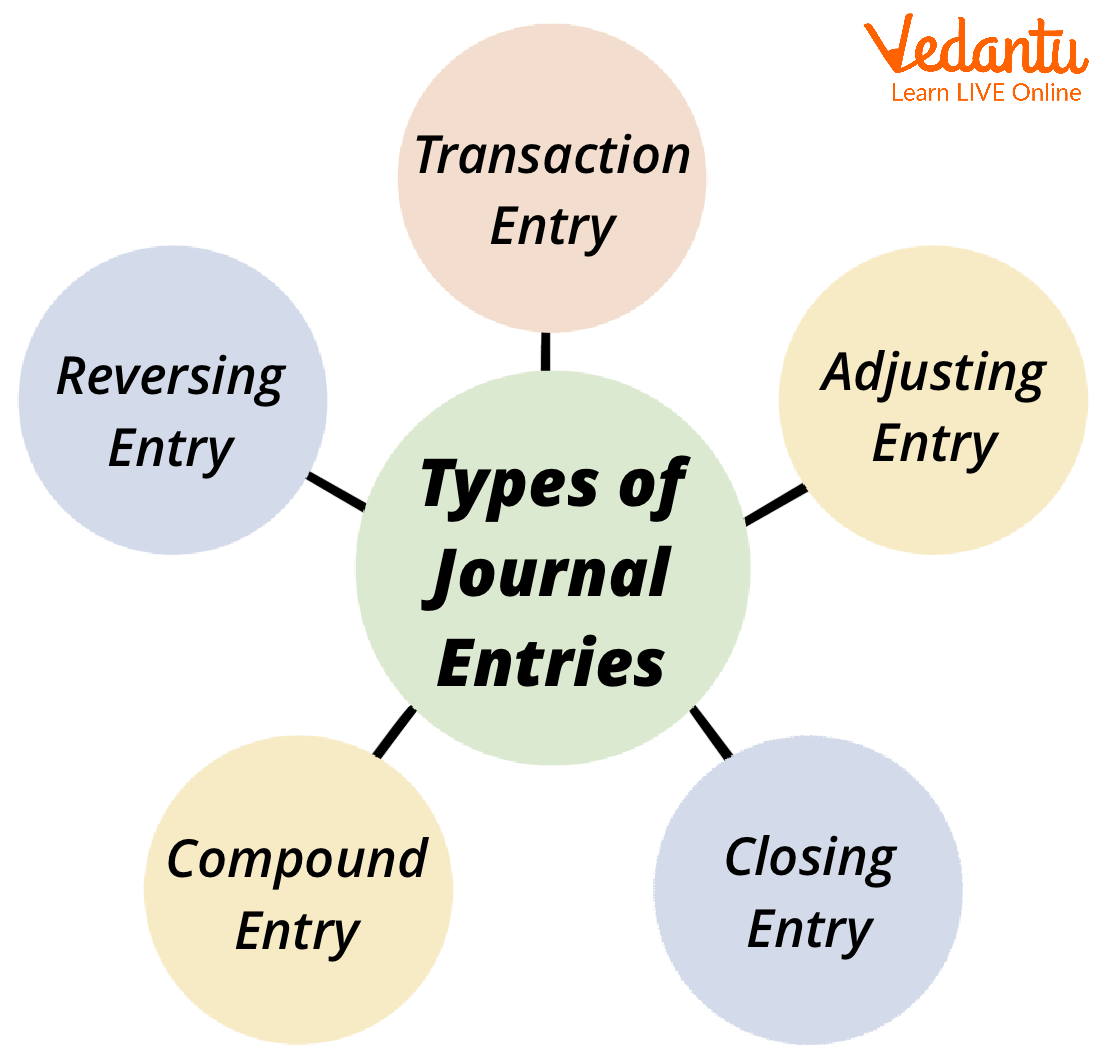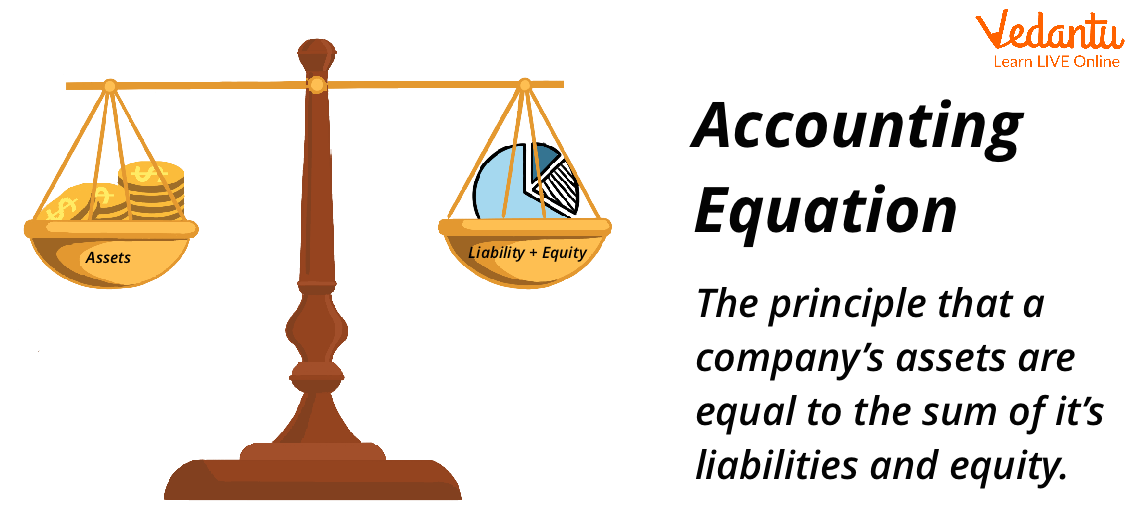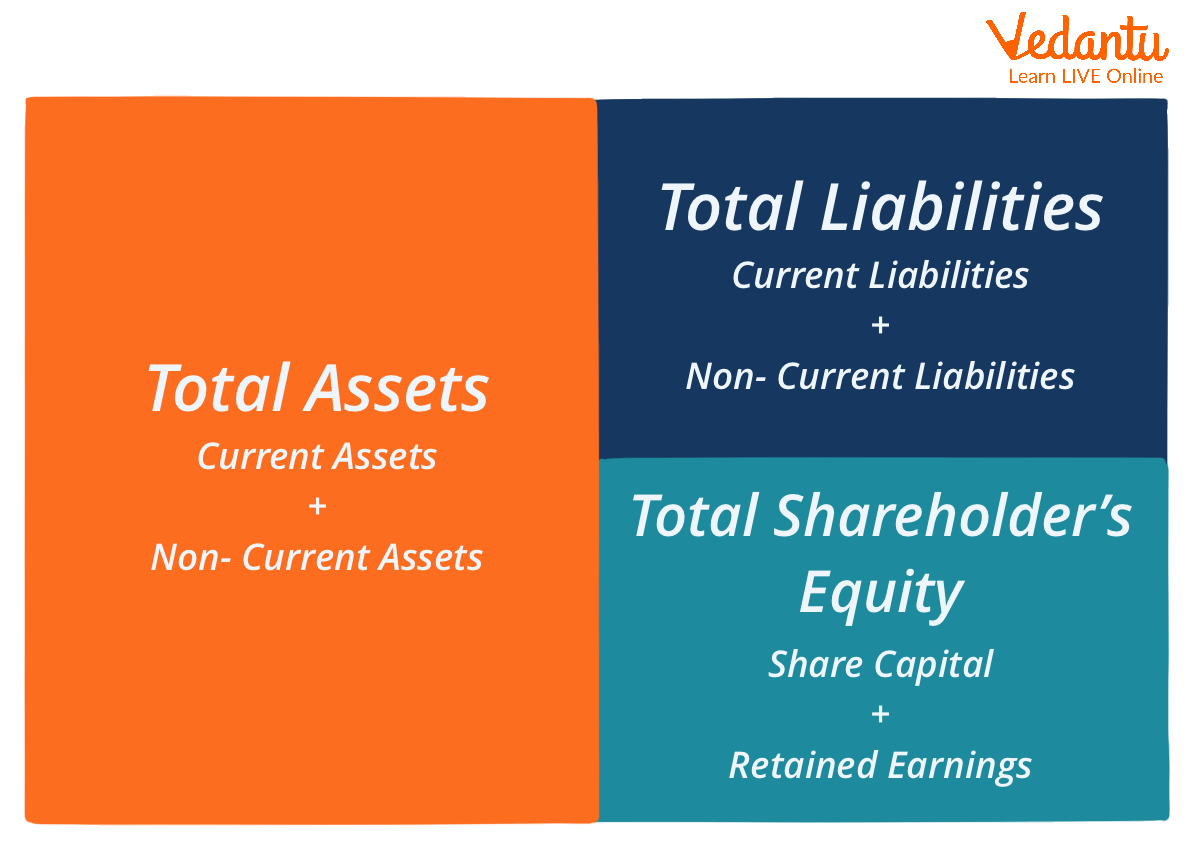Courses
Courses for Kids
Free study material
Free LIVE classes
More

# Journal Entry through Accounting EquationLIVE
Join Vedantu’s FREE Mastercalss

## Introduction of Journal Entry

Journal entries are the initial stage in the accounting process. The procedure entails reviewing business transactions to see if they have an economic effect on the company's accounts. This process begins at the start of the accounting cycle and continues throughout the period.

After determining whether transactions impact the economy, the bookkeeper will record these transactions in the journal entry. A journal entry is a daybook or master diary that records all business transactions within an accounting cycle.Types of Journal Entries

## What is the Accounting Equation?

The accounting equation is a fundamental accounting principle and a key component of the balance sheet. The formula is as follows:

Assets = Liabilities + Shareholder’s Equity.

This equation establishes the cornerstone of double-entry accounting, often called double-entry accounting, and shows the balance sheet structure. Every transaction in double-entry accounting impacts at least one or two accounts.

An increase in an asset account, for example, can be matched by an equivalent rise in a corresponding liability or shareholder's equity account, ensuring that the accounting equation remains balanced. Alternatively, a rise in one asset account might be offset by a reduction in another. When conducting journal entries, it is critical to remember the accounting equation.Accounting Equation Concept

## How can this Equation Work?

The essential accounting system (Assets = Liability + Shareholders' Equity) is lengthened by the extended accounting system. The formula displays elements from the balance sheet's shareholders' Equity section.

The expanded accounting equation is:

Total Assets = Total Liabilities + CC +/- AOCIL + BRE + R – E – D – SR.

Where terms from the Shareholder’s Equity section of the balance sheet include:

• CC is Contributed Capital

• AOCIL is Accumulated Other Comprehensive Income (Loss)

• BRE is Beginning Retained Earnings

• R is Revenue

• E is Expenses

• D is Dividends (paid)

• SR is Stock Repurchases

If the assets are not retired, they are referred to as treasury stock. Treasury stock purchases and sales are reported in retained profits and paid-in capital. The specifics of the transaction determine the journal entry.

## Accounting Equation Formula

Accounting equation formula: Assets=(Liabilities + Owner’s Equity). As an illustration, suppose the prominent retail XYZ Corporation recorded the following on its income statement for its most recent full fiscal year:

• Total assets: \$170 billion

• Total Liabilities: \$120 billion

• Total shareholders' equity: \$50 billion

If we compute the right-hand side of the financial statement (equity + liabilities), we get (\$ 50 billion + \$120 billion) = \$170 billion, which corresponds to the value of the company's assets.Accounting Equation Formula

## Accounting calculations

This equation is based upon the double-entry bookkeeping method, which implies that all assets in the book of accounts ought to equal all liabilities. A matching credit entry should accompany every item made to the balance sheet's debit side. As a result, it's also known as the balance sheet equation. A balance sheet equation can be represented as:

Total Assets = Total Liabilities + Total Shareholder’s Equity

## Rules of Accounting Equation

• Capital: Capital increases are recorded as credits, while capital withdrawals are recorded as debits.

• Profit and, by extension, capital are increased by the interest paid on the former. Owner losses and expenses reduce available funds.

• The interest earned on invested money and interest earned on withdrawal will not be shown in the balance sheet as either an asset or a liability.

• Revenue: The revenue increases the owner’s equity/capital.

• Expenses: The owner’s equity/capital is decreased by the number of expenses.

• Owners’ Equity: Any rise in obligations results in a credit to the liability equity held by outsiders, whereas any reduction in liabilities results in a debit.

• The asset account is credited if there is a rise in assets. The asset account is debited if there is a drop in assets.

## Conclusion

The accounting equation displays the institution's valuable resources as obligations in the form of liabilities, assisting shareholders in determining the company's worth and establishing the link between them.

The accounting equation assures that the balance sheet is always balanced. For every debit entry, there is an equal credit entry (or coverage). Accounting equations are often known as fundamental accounting equations or balance sheet equations. Calculating the credits and debits of commercial transactions helps in preserving business efficiency.

Last updated date: 25th Sep 2023
Total views: 95.1k
Views today: 1.95k

## FAQs on Journal Entry through Accounting Equation

1. What are the general rules for the accounting equation?

It has the following formula: Assets = Liabilities + Owner's Equity. For every transaction in a business, there is a balance that is happening between the three elements of the accounting equation. Assets will always equal the sum of liabilities and owner's equity. Assets are everything that a company owns. In other words, an accounting system should always equal the total amount of debits and credits. The accounting equation assists in maintaining accuracy for accountants and the accounting profession.

2. How can we apply the accounting equation in our daily lives?

Accounting is essential for all types of entities to operate. Every day people like us use it regularly. We use bookkeeping to handle our bills, monitor our money, and buy groceries.

• Financial planning or budgeting. The majority of businesses employ accountants at the start of each fiscal year to advise them on how to allocate their funds effectively.

• Tracking your expense and income

• Saving for the future

• Investing to make more money

3. How does the accounting equation relate to the income statement?

Balance Sheet and Income Statement
Like the accounting equation, it shows that a company's total amount of assets equals the total amount of liabilities plus the owner's (or stockholders') equity. The income statement is the financial statement that reports a company's revenues and expenses and the resulting net income. A balance sheet displays the financial situation of the firm at a certain point in time, whereas an income statement analyses a company's profit or loss over a specified period.